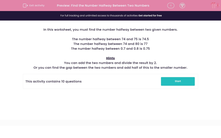# Find the Number Halfway Between Two Numbers

In this worksheet, students find the number halfway between two given numbers.This content is premium and exclusive to EdPlace subscribers.Key stage:  KS 2

Curriculum topic:   Maths and Numerical Reasoning

Curriculum subtopic:   Place Value

Difficulty level:#### Worksheet Overview

In this worksheet, you must find the number halfway between two given numbers.

The number halfway between 74 and 75 is 74.5

The number halfway between 74 and 80 is 77

The number halfway between 0.7 and 0.8 is 0.75

Hints

You can add the two numbers and divide the result by 2.
Or you can find the gap between the two numbers and add half of this to the smaller number.

### What is EdPlace?

We're your National Curriculum aligned online education content provider helping each child succeed in English, maths and science from year 1 to GCSE. With an EdPlace account you’ll be able to track and measure progress, helping each child achieve their best. We build confidence and attainment by personalising each child’s learning at a level that suits them.

Get started